## Facebook Hacker Cup 2016: Round 2 problem analysis

Yesterday Round 2 of the Facebook Hacker Cup was held. This round lasted only 3 hours, and competition was fierce. Only 200 of over 2000 competitors would advance. In the end, over 177 competitors solved all problems. To advance without a perfect score, you could only fail on the easiest problem, and you'd have to submit the other solutions pretty quickly, too.

Unfortunately, under time pressure, I made a small mistake in my solution to the fourth problem, and so I won't be participating in Round 3 this year.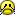On the bright side, at least I'll get a nice T-shirt for my troubles. And you get to read another one of my write-ups!

#### Problem A: Boomerang Decoration (15 points)

Official problem statement

The first problem is an ad-hoc problem with a somewhat silly description. Effectively, we're asked how many operations are needed to transform a string A into a string B, given that each operation consists of overwriting a prefix of a certain length with one character, and at the same time overwriting a suffix with another letter.

To attack this problem, it makes sense to start by breaking up the destination string, B, into same-colored segments, because we should clearly be covering these in a single operation. Changing "XXX" to "ABC" is effectively the same as changing "XXXXXX" to "ABBCCC": it's the color changes that matter, not the length of the segments of a single color. Additionally, it's useful to know for each segment of B whether or not it's equal to the corresponding segment in A, because if it is, we might not need to repaint it at all (for example, when transforming from "ABC" to "CBA", we can leave the middle segment unchanged.)

After this, we can view the list of segments as a binary string where each bit indicates wheter the corresponding segment of B was equal to that in B. For example, "ABC" -> "CBA" corresponds with "101", and so does "AABBCC" -> "CCBBAA", and "FOXENRULE" -> "NOREAALLY" corresponds with "1010111". The question is then: how can we split that binary string into three parts (corresponding with the prefix painted by Jack, the middle part not painted at all, and the suffix painted by Jill) so that the middle part is all-zeroes, and the maximum of the prefix and suffix length is minimal?

To answer that, we can consider every position where a '1' appears, from left to right. If that's the last position painted by Jack, then we can skip all zeroes to the right (the middle part that won't be painted) until we find the next '1', which must be painted by Jill. The total time taken in that case is the maximum of the prefix size and suffix size. For the final answer, we just take the minimum over all possibilities.

My solution looked roughly like this:
Python:
 ```1 2 3 4 5 6 7 8 9 10 11 12 13 14 15 16 17 18 19 20 21 22 23 24 25 26 27 28 29 30 ``` ```from sys import stdin def GetSegments(N, A, B): segments = [] i = 0 while i < N: j = i + 1 while j < N and B[i] == B[j]: j += 1 segments.append(A[i:j] != B[i:j]) i = j return segments def Solve(segments): M = len(segments) i = -1 answer = M while i < M: j = i + 1 while j < M and not segments[j]: j += 1 answer = min(answer, max(i + 1, M - j)) i = j return answer for case in range(1, int(stdin.readline()) + 1): N = int(stdin.readline()) A = stdin.readline().strip() B = stdin.readline().strip() print('Case #{}: {}'.format(case, Solve(GetSegments(N, A, B))))```

The only tricky part in this problem is that the input strings can be fairly long (up to 100,000 characters), so we must make sure that we don't write a quadratic-time solution by mistake. That's why Solve() skips from 1 to 1 in the outer loop, while skipping over the 0s in the inner loop. That way, we'll only look at each segment once.

#### Problem B: Carnival Coins (20 points)

Official problem statement

The second problem is a combination of basic probability theory, and dynamic programming. To solve it, we need to define two recursive functions. First, for a fixed P, what's the probability that flipping some n coins will give us at least k heads? If k=0, then the probability is 1 (we don't need any heads!) Similarly, if n<k, then the probability is 0 (because each coin can only come up heads, once). In particular, this covers the case where n=0. For other values of n and k, we can simply start by flipping one coin: either it will come up heads (with probability P) and then we need (k-1) more heads, or it comes up tails (with probability 1-P) and we still need k more heads. Either way, we'll have n-1 unflipped coins left.

To write this down, formally:
```Chance(n,k) = 1  (if k = 0)
Chance(n,k) = 0  (if n < k)
Chance(n,k) = Chance(n-1, k-1)*P + Chance(n-1, k)*(1 - P)  (if 0 < k <= n)
```

The probability of winning a prize using n coins is simply Chance(n, K) (where K is the required number of heads which is fixed for the problem). That's the probability for just one prize. What is the expected value for a total of N coins? We can calculate that in a slightly different way: if we have no coins, than we will win no prizes. Otherwise, let's say we have n coins, and we use anywhere between 1 and n coins to flip, to try and win one more prize; let's call that number m. The expected value in that case is equal to the chance of winning one prize with our m coins, plus the expected number of prizes we can win with the remaining (n-m) coins. Formally:
```Prizes(n) = 0  (if n = 0)
Prizes(n) = maximum of Chance(m, K) + Prizes(n - m) for 1 <= m <= n  (if n > 0)
```

Now we simply want to know the expected number of prizes if we start with N coins: that is, Prizes(N). Coding it up:
Python:
 ```1 2 3 4 5 6 7 8 9 10 11 12 13 14 15 16 17 18 19 20 21 22 ``` ```from sys import stdin def Solve(N, K, P): Chance = {} for n in range(N + 1): for k in range(K + 1): Chance[n, k] = ( 1 if k == 0 else 0 if n < k else Chance[n - 1, k - 1]*P + Chance[n - 1, k]*(1 - P)) Prizes = {} for n in range(N + 1): Prizes[n] = ( 0 if n == 0 else max(Chance[m, K] + Prizes[n - m] for m in range(1, n + 1))) return Prizes[N] for case in range(1, int(stdin.readline()) + 1): N, K, P = stdin.readline().split() print('Case #{}: {:.9f}'.format(case, Solve(int(N), int(K), float(P))))```

This solution is a little on the slow side (it takes about 2 minutes on the official data set) but it should illustrate the right idea. If you've been reading my write-ups of earlier rounds, you may notice a pattern: many contests include a dynamic programming problem, and all of them have very straight-forward code. In these cases, it's a good idea to work out the details on paper before you start coding. Once you're convinced that you've got the functions defined correctly, the implementation is trivial.

#### Problem C: Snakes and Ladders (25 points)

Official problem statement

Problem C requires some mathematical tricks. For one thing, we are asked to calculate the answer modulo 1,000,000,007. This has little to do with the actual problem; it's just a way to keep the output reasonably small, and to make sure that it can be calculated using just signed 32-bit integers (which have a range a little over 2 billion). If you don't know anything about modular arithmetic, you should read up on it at some point. For this problem, it's enough to know that all additions, subtractions and multiplications must be performed modulo M = 1,000,000,007.

The hardest part of solving problem C is actually a more specific subproblem: what if all the ladders have the same height? In that case, there is a snake between every pair of ladders. Effectively, we have a list of increasing x-coordinates, and we want to calculate the sum of the squares of all differences between pairs. The simplest way to do that, would be something like this:
Python:
 ```1 2 3 4 5 6 7 ``` ```def Cost1(xs): res = 0 for i in range(len(xs)): for j in range(i + 1, len(xs)): d = xs[j] - xs[i] res += d*d return res```

This looks correct, but if we check the constraints for the problem statement, we see that we could have up to N=200,000 ladders for a single testcase, and these could all have the same height. In that case, our O(N*N) solution will be too slow. To speed it up, let's apply some calculcus: the derivative of x2 = 2x. In other words, the difference between squares increases linearly. It's more obvious if we write down the first few squares and their differences:
x012345
x201491625
(x+1)2 - x21357911

Note that the squares in the middle row are the sum of the middle and bottom cell of the previous column (e.g. 25 = 16+9). That means we can step through the table from left to right and update the squares using only additions:
Python:
 ```1 2 3 4 5 6 7 8 9 10 11 ``` ```def Cost2(xs): res = 0 for i in range(len(xs)): x, y = 0, 1 for j in range(i + 1, len(xs)): d = xs[j] - xs[i] for _ in range(d): x += y y += 2 res += x return res```

At this point you might think "Wow, thanks a lot, Soultaker! This is even slower!" and you would be right. This doesn't even pass the sample input, because we step through the squares one by one, so going from x=1 to x=1,000,000,000 takes 1,000,000,000 steps. So let's fix that. Since we know that we will add 2 to y, d times, we might as well add 2d to y outside the loop.

And since we know that we add y to x, d times, we can simply multiply the average value of y by d, and add that to x. Note that the first value is simply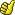and the last value is (y + 2*(d - 1)), so the average is (y + (y + 2*(d - 1))/2, which simplifies to (y + d - 1) (really, try it yourself if you don't believe me):
Python:
 ```1 2 3 4 5 6 7 8 9 10 ``` ```def Cost3(xs): res = 0 for i in range(len(xs)): x, y = 0, 1 for j in range(i + 1, len(xs)): d = xs[j] - xs[j - 1] x += d*(y + d - 1) y += 2*d res += x return res```

Now we can solve the sample case again. Although it may seem we haven't made much progress, the code is in just the right shape to apply a final trick. Note that for each value of j, the difference between xs[j] and xs[j - 1] is the same for all possible values of i < j. That means we can combine those, and update the value of y at once for all preceding i's. And if we do that, instead of calculating the average y for one fixed i, we can calculate the average over all y:
Python:
 ```1 2 3 4 5 6 7 8 9 ``` ```def Cost4(xs): res = 0 x, y = 0, 1 for j in range(1, len(xs)): d = xs[j] - xs[j - 1] x += d*y + d*(d - 1)*j y += 2*d*j + 1 res += x return res```

Finally! A linear time solution for that nasty subproblem. This transformation was pretty tricky, which is why I listed all the intermediate steps. If you're still confused, just start with Cost1() at the top, and check that each following implementation is equivalent to the one before it.

Of course, we're not done yet. Our final Cost() function calculates the sum of squares of distances between all pairs of ladders of the same height. We still need to generalize to the case where there are ladders of different heights.

It turns out that this part is relatively simple. Snakes occur in groups of ladders of the same height, which are separated by ladders of larger heights. For example, if the heights (ignoring the coordinates) of the ladders in the input from left-to-right are: 1,1,9,1,1,9,1,1, then there is one snake between the pair of ladders of height 9, and one snake between each pair of ladders of height 1. That means that if we sort the ladders by x-coordinate and we go through them from left to right, then if the next ladder has height h, we can immediately collect the groups of ladders to the left with height less than h. For each group, we take the x-coordinates, and calculate the cost as defined above.

Remember that we'll also have to add some modulo-operations at strategic locations to prevent our intermediate results from growing too large. The final solution looks something like this:
Python:
 ```1 2 3 4 5 6 7 8 9 10 11 12 13 14 15 16 17 18 19 20 21 22 23 24 25 26 27 28 29 30 31 32 33 34 35 36 37 38 39 40 ``` ```from sys import stdin M = 1000000007 def Cost(xs): res = 0 x, y = 0, 1 for j in range(1, len(xs)): d = xs[j] - xs[j - 1] x += d*y + d*(d - 1)*j x %= M y += 2*d*j + 1 y %= M res += x res %= M return res def Solve(ladders): ladders.sort() ladders.append((max(x for (x,h) in ladders) + 1, max(h for (x,h) in ladders) + 1)) answer = 0 previous = [] # list of (height, list-of-xs) of previous ladders for x, h in ladders: while previous and previous[-1] < h: answer += Cost(previous.pop()) answer %= M if previous and previous[-1] == h: previous[-1].append(x) # append to existing group of height h else: previous.append((h, [x])) # start a new group of height h return answer for case in range(1, int(stdin.readline()) + 1): N = int(stdin.readline()) ladders = [] for n in range(N): x, h = map(int, stdin.readline().split()) ladders.append((x, h)) print('Case #{}: {}'.format(case, Solve(ladders)))```

Note that after sorting the ladders, I add a "dummy ladder" at the end of the list, which is higher and farther away than any other ladder. The purpose of this is to make sure that all groups of ladders to its left have been processed at the end of the loop. (There are other ways to achieve the same effect, of course.)

#### Problem D: Costly Labels (40 points)

Official problem statement

The fourth and final problem was not as difficult as you might expect based on its point value. The trick is to realize that although the tree can be fairly large (up to 1,000 nodes) the number of colors is fairly small (up to 30).

If we traverse the tree from root to leafs (we can take any node as the root; I simply chose the first one) then for every node, we have the choice: either we accept the penalty P, and then we don't care what the colors of our neighbours are, or we avoid the penalty, and then we must make sure all our neighbours have different colors. Note that "neighbours" here means just "children" plus exactly one "parent" (except for the root, which has no parent). If the number of neighbouring nodes is larger than K, then it's not possible to assign them all a different color (by the pigeonhole principle).

Putting these two options together, we can solve the problem recursively as follows. If we arrive at a node v, and we know the color of our parent, then we can pick any color for v, and calculate for each child what the cost of labelling the subtree in different colors would be. After all, the cost of labeling a subtree only depends on the color of its parent. Then, we have a CxK matrix, where C is the number of children, K is the number of colors, and the value at row i, column j equals the cost of coloring the i-th child with the j-th color. If we choose to incur the penalty, then for each child, we can take the minimum cost regardless of color, add them up, and add the penalty P. That's option 1.

If the number of children is small enough (C < K, or C <= K at the root) then we can try to assign them all different colors to avoid the penalty. That's option 2. Effectively, we want to pick C cells in our matrix such that each selected cell is in a different row and column (i.e. the assignment of colors to nodes is unique). Additionally, we can't pick the column that corresponds with the color of our parent (if any). This is an instance of the well-known assignment problem. The typical algorithm to solve these problems is called the Hungarian algorithm after its inventors from the 1950s, but I chose to solve it as a minimum-cost flow problem instead, because I had library code ready for this.

The complete solution goes through the tree from top to bottom, making sure not to calculate the solution for every combination of vertex, color and parent's color) only once, and deciding the minimum cost for this combination by taking the cheapest of the 2 options described above.

Since there are N*K*K combinations, and solving the assignment problem for a K*K matrix takes O(K3) time, that would lead to an O(N*K5) solution. However, not all vertices can have K neighbours; in the worst case there are N/K, which means we can remove one factor of K to have an O(N*K4) solution. Again, the fact that K is reasonably small means that this is just good enough to work.

Since my solution code is quite a bit longer than usual, I won't post it inline, but I've posted it to Github instead: D.cc. In case anyone is curious why this solution didn't work during the contest: I forgot to add the m = part on line 124. I'll leave it as an exercise to the reader to figure out why that bit is important.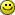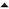09-'17 Temporarily disabling Address Space Layout Randomization on Linux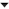01-'16 Facebook Hacker Cup 2016: Round 1 problem analysis

#### Reacties

Thanks for doing this:-) I like it when people get into the details!

Reageren is niet meer mogelijk